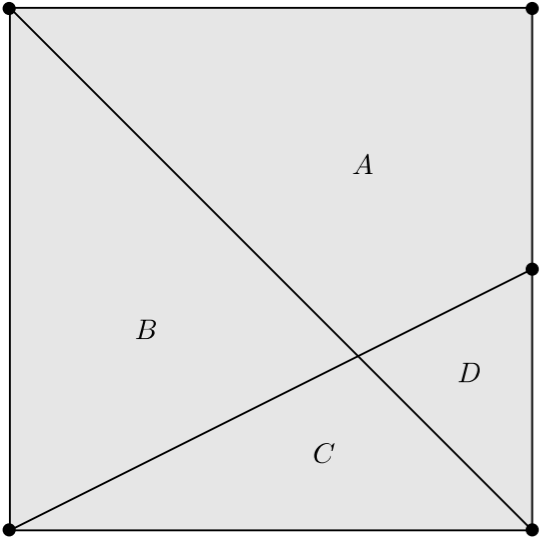# Square Division

Geometry Level 4A diagonal is constructed in a square.

On the other pair of corners, a line is drawn from one of the two such that it intersects the midpoint of one of the opposite sides.

These two lines divide the square into four regions, $A$, $B$, $C$, $D$, in order from largest area to smallest area.

The ratio of these four areas can be expressed as $a:b:c:d$ where $a$, $b$, $c$, $d$, are all integers and are setwise coprime, but not necessarily pairwise coprime.

Find $a+b+c+d$

×